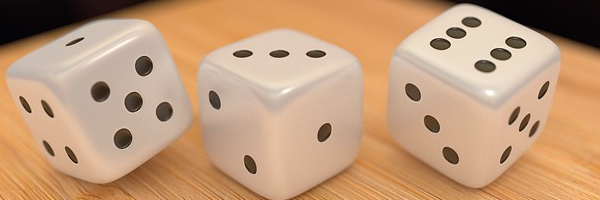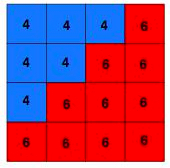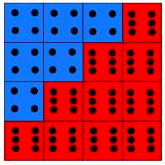# Dice in a SquareWe have 6 blue dice with 4's on top in a triangle joined with 10 red dice with 6's on top also in a triangle, making a square.or asAdding up gives a total of 84 - check that! How did you count?

There's a rule for how these are set up - the blue dice have to have the same numbers on the top and the red dice have to use a different number to the blue, but the same on each.

The kind of questions we might ask are;

What other totals can you make?
How many different arrangements can be made?
What if the two triangles (that together make up the square) were a different size giving a total of 9, 25, 36 . . . . . dice?